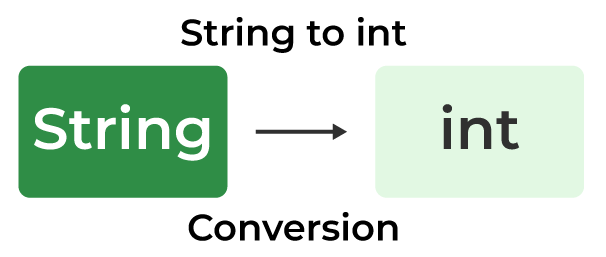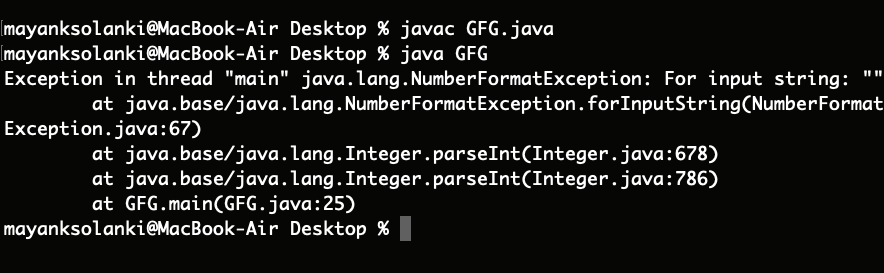Open In App

# Java Program to Convert a String to Int

In Java, while operating upon strings, there are times when we need to convert a number represented as a string into an integer type. We usually do this because we can operate with a wide flexible set of operations over strings. The method generally used to convert String to Integer in Java is parseInt() of the String class. In this article, we will check on Java String to Int conversion.## Methods to convert Java String in int

There are two basic well-known methods to convert string to int java as mentioned below:

• Using ParseInt() method
• Using valueOf()

## 1. parseInt() Method in Java

### Variants of parseInt Java Method

There are two variants of this method:

1. parseInt(String s): This function parses the string argument as a signed decimal integer.
2. parseInt(String s, int radix): This function parses the string argument as a signed integer in the radix specified by the second argument.

#### Syntax of parseInt

```public static int parseInt(String s);         throws NumberFormatException
public static int parseInt(String s, int radix);         throws NumberFormatException```

#### Parameters

• A string that needs to be converted to an integer. It can also have the first character as a minus sign ‘-‘ (‘\u002D’) or plus sign ‘+’ (‘\u002B’) to represent the sign of the number.
• The radix is used while the string is being parsed.

This parameter is only specific to the second variant of the method.

#### Return Type

Both methods convert the string into its integer equivalent. The only difference is that of the parameter radix. The first method can be considered as an equivalent of the second method with radix = 10 (Decimal).

#### Exception Thrown

NumberFormatException is thrown by this method if any of the following situations occur.

Remember certain key points associated with both variants:

1. The string is null or of zero-length
2. The value represented by the string is not a value of type int
3. Specifically for the parseInt(String s, int radix) variant of the function:
• Any character of the string is not a digit of the specified radix, except that the first character may be a minus sign ‘-‘ (‘\u002D’) or plus sign ‘+’ (‘\u002B’) provided that the string is longer than length 1
4. If your String has leading zeroes, the parseInt() method will ignore them.

### Example of Java parseInt() Method

#### Code:

```String str=”0111”;
int t=Integer.parseInt(str);
System.out.println(t); ```

#### Output:

`111`

For the above code, the snippet output is 111. So, in the cases where leading zeroes are important(in binary representations) avoid using the parseInt() method.

### Illustrations of parseInt() Method

Let’s take a random code snippet further understand the illustrations better.

#### 1.  Passing a String

```parseInt("20") returns 20
parseInt("+20") returns 20
parseInt("-20") returns -20```

#### 2. Passing a String and an Integer

```parseInt("20", 16) returns 16, (2)*16^1 + (0)*16^0 = 32
parseInt("2147483648", 10) throws a NumberFormatException
parseInt("99", 8) throws a NumberFormatException
as 9 is not an accepted digit of octal number system
parseInt("geeks", 28) throws a NumberFormatException
parseInt("geeks", 29) returns 11670324,
Number system with base 29 can have digits 0-9
followed by characters a,b,c... upto s

parseInt("geeksforgeeks", 29) throws a NumberFormatException as the
result is not an integer.```

## How to use the parseInt() Method?

Let us take an example straight away in order to get used so do the working of parseint() method and implement the above-mentioned as follows:

## Java

 `// Java program to demonstrate working parseInt()``// Where No NumberFormatException is Thrown`` ` `// Main class``public` `class` `GFG {`` ` `    ``// Main driver method``    ``public` `static` `void` `main(String args[])``    ``{``        ``// Custom wide-varied inputs to illustrate``        ``// usage of valueOf() method``        ``int` `decimalExample = Integer.parseInt(``"20"``);``        ``int` `signedPositiveExample = Integer.parseInt(``"+20"``);``        ``int` `signedNegativeExample = Integer.parseInt(``"-20"``);``        ``int` `radixExample = Integer.parseInt(``"20"``, ``16``);``        ``int` `stringExample = Integer.parseInt(``"geeks"``, ``29``);`` ` `        ``// Print commands on console``        ``System.out.println(decimalExample);``        ``System.out.println(signedPositiveExample);``        ``System.out.println(signedNegativeExample);``        ``System.out.println(radixExample);``        ``System.out.println(stringExample);``    ``}``}`

Output

```20
20
-20
32
11670324
```

## Java

 `// Java Program to Demonstrate Working of parseInt() Method``// Where NumberFormatException is Thrown`` ` `// Main class``public` `class` `GFG {`` ` `    ``// Main driver method``    ``public` `static` `void` `main(String args[])``    ``{``        ``// Custom wide-varied inputs to illustrate``        ``// usage of valueOf() method``        ``int` `decimalExample = Integer.parseInt(``"20"``);``        ``int` `signedPositiveExample = Integer.parseInt(``"+20"``);``        ``int` `signedNegativeExample = Integer.parseInt(``"-20"``);``        ``int` `radixExample = Integer.parseInt(``"20"``, ``16``);``        ``int` `stringExample = Integer.parseInt(``"geeks"``, ``29``);`` ` `        ``// It will raise NumberFormatException``        ``String invalidArguments = ``""``;``        ``int` `emptyString``            ``= Integer.parseInt(invalidArguments);``        ``int` `outOfRangeOfInteger``            ``= Integer.parseInt(``"geeksforgeeks"``, ``29``);``        ``int` `domainOfNumberSystem``            ``= Integer.parseInt(``"geeks"``, ``28``);`` ` `        ``// Print commands on console``        ``System.out.println(decimalExample);``        ``System.out.println(signedPositiveExample);``        ``System.out.println(signedNegativeExample);``        ``System.out.println(radixExample);``        ``System.out.println(stringExample);``    ``}``}`

#### OutputSimilarly, we can convert the string to any other primitive data type:

1. parseLong(): parses the string to Long
2. parseDouble(): parses the string to double
If we want to convert the string to an integer without using parseInt(), we can use valueOf() method. It also has two variants similar to parseInt()
3. Difference between parseInt() and valueOf(): parseInt() parses the string and returns the primitive integer type. However, valueOf() returns an Integer object.

Note: valueOf() uses parseInt() internally to convert to integer.

## 2. Using valueOf() Method to Convert String to Int Java

The Integer.valueOf() method converts a String into an Integer object.

Below is the implementation of the above method:

## Java

 `// Java Program to Demonstrate``// Working of valueOf() Method`` ` `// Main class``public` `class` `GFG {``    ``// Main driver method``    ``public` `static` `void` `main(String args[])``    ``{``        ``// Custom wide-varied inputs to illustrate``        ``// usage of valueOf() method``        ``int` `decimalExample = Integer.valueOf(``"20"``);``        ``int` `signedPositiveExample = Integer.valueOf(``"+20"``);``        ``int` `signedNegativeExample = Integer.valueOf(``"-20"``);``        ``int` `radixExample = Integer.valueOf(``"20"``, ``16``);``        ``int` `stringExample = Integer.valueOf(``"geeks"``, ``29``);`` ` `        ``// Print statements``        ``System.out.println(decimalExample);``        ``System.out.println(signedPositiveExample);``        ``System.out.println(signedNegativeExample);``        ``System.out.println(radixExample);``        ``System.out.println(stringExample);``    ``}``}`

Output

```20
20
-20
32
11670324
```